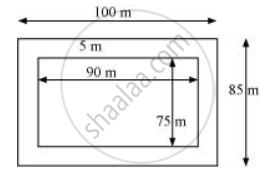# A Garden is 90 M Long and 75 M Broad. a Path 5 M Wide is to Be Built Outside and Around It. Find the Area of the Path. Also Find the Area of the Garden in Hectare - Mathematics

A garden is 90 m long and 75 m broad. A path 5 m wide is to be built outside and around it. Find the area of the path. Also find the area of the garden in hectare

#### SolutionLength (l) of garden = 90 m

Breadth (b) of garden = 75 m

Area of garden = l × b = 90 × 75 = 6750 m2

From the figure, it can be observed that the new length and breadth of the garden, when path is also included, are 100m and 85m respectively.

Area of the garden including the path = 100 × 85 = 8500 m2

Area of path = Area of the garden including the path − Area of garden

= 8500 − 6750 = 1750 m2

1 hectare = 10000 m2

Therefore, area of garden in hectare = 6750/10000 = 0.675 hectare

Concept: Conversion of Units
Is there an error in this question or solution?

#### APPEARS IN

NCERT Class 7 Maths
Chapter 11 Perimeter and Area
Exercise 11.4 | Q 1 | Page 226